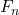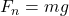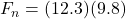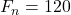## Calculate the force of kinetic friction on a 12.3 kg mass if its coefficient of kinetic friction is 0.137

Question

Calculate the force of kinetic friction on a 12.3 kg mass if its coefficient of kinetic friction is 0.137

in progress 0
2 months 2021-07-30T09:48:44+00:00 1 Answers 0 views 0

Explanation:

All we need here is the equation for frictional force, which is

f = μwhereThat’s normal force which is the same thing as weight.

Therefore,to 2 sig figs soand

f = (.137)(12) to 2 sig figs as well so

f = 16N